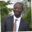Related Tags

javascript
communitycreator

# How to get the sum of an array in JavaScriptAbel Lifaefi Mbula

In this shot, we will discuss three methods you can use to get the total of a given array in JavaScript.

First, we’ll use the traditional for loop. Secondly, we’ll use forEach, an array-like method, and lastly, we’ll make use of for...of.

In this shot, our array example is [1, 4, 0, 9, -3], and the expected output is 11.

## 1. Using the traditional for loop

In this method, you iterate and add each item until you reach the last item.

function sumArray(array){
let sum = 0 // the sum is initialed to 0

/* js arrays are zero-index based
ourArray.length = 5, the initialization block is set to 0.
the last item is index 4 that is < 5 (what we define in the condition block)
*/
for (let i = 0; i <
array.length; i += 1) {
// take every item in the array and add it to sum variable
sum += array[i]
// initial: sum = 0
// iteration 1: 0 + 1 => sum = 1
// iteration 2: 1 + 4 => sum = 5
// iteration 3: 5 + 0 => sum = 5
// iteration 4: 5 + 9 => sum = 14
// iteration 5: 14 + -3 => sum = 11

}

console.log(sum) // 11
// return sum
return sum
}

// call the function and give it our array
sumArray([1, 4, 0, 9, -3]); // logs 11


function sumArray(array) {
const ourArray = [1, 4, 0, 9, -3];
let sum = 0;

for (let i = 0; i < ourArray.length; i += 1) {
sum += ourArray[i];
}

return sum;
}

console.log(sumArray([1, 4, 0, 9, -3]));

## 2. Using forEach

forEach is a built-in or array-like method. It’s simple, as shown below.

function sumArray(array) {
let sum = 0;

/*Go through each item in the array and execute this function which adds
each item to sum
*/
array.forEach(item => {
sum += item;
});

console.log(sum);
return sum;
}

sumArray([1, 4, 0, 9, -3]); //logs 11


As you can see, the solution is short and clean.

function sumArray(array) {
let sum = 0;

array.forEach(item => {
sum += item;
});

console.log(sum);
return sum;
}

sumArray([1, 4, 0, 9, -3]);

## 3. Using for...of

for...of lets us iterate over an array, so we can make use of it to get the sum of an array. Since we keep the same style of functions as above, let’s copy and adjust.

function sumArray(array) {
let sum = 0;

/*loop over array and add each item to sum
*/
for (const item of array) {
sum += item;
}

// return the result
console.log(sum);
return sum;
}

sumArray([1, 4, 0, 9, -3]); //logs 11


We still get the same result as the other methods we’ve used. You can test it out on the widget below.

function sumArray(array) {
let sum = 0;

/*loop over array and add each item to sum
*/
for (const item of array) {
sum += item;
}

// return the result
console.log(sum);
return sum;
}

sumArray([1, 4, 0, 9, -3]); //logs 11

RELATED TAGS

javascript
communitycreator

CONTRIBUTORAbel Lifaefi Mbula
RELATED COURSES

View all Courses

Keep Exploring

Learn in-demand tech skills in half the time